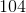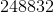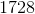### Test: Computer Science

Consider the following code:

public static void main(String[] args) {

System.out.println(equation(8));

}

public static int equation(int a) {

if(a <= 5) {

return 12;

}

return equation(a-2) * equation(a-1);

}

 1 What is the output for the code above?1/3 questions

0%

## Access results and powerful study features!

Take 15 seconds to create an account.